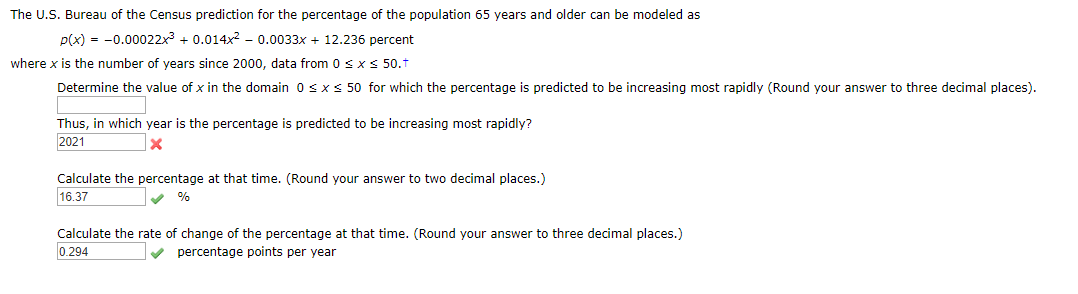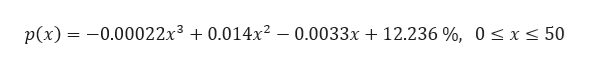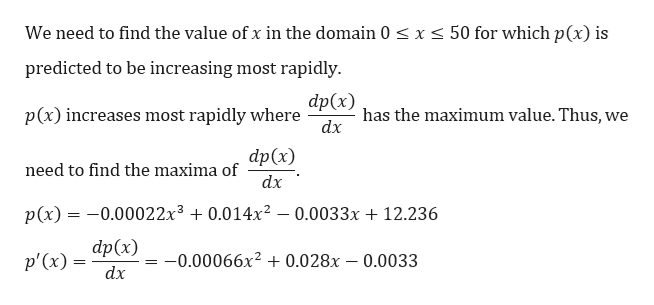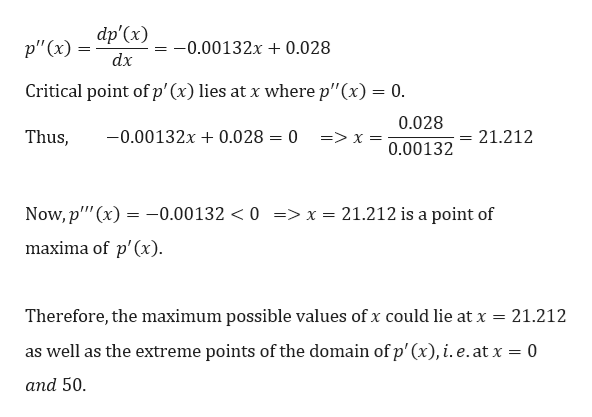# The U.S. Bureau of the Census prediction for the percentage of the population 65 years and ol der can be modeled asp(x)-0.0002 2x3 0.014x2 - 0.0033x + 12.236 percentwhere x is the number of years since 2000, data from 0 s x S 50.Determine the value of x in the domain 0 s x50 for which the percentage is predicted to be increasing most rapidly (Round your answer to three decimal places)Thus, in which year is the percentage is predicted to be increasing most rapidly?2021XCalculate the percentage at that time. (Round your answer to two decimal places.)16.37%Calculate the rate of change of the percentage at that time. (Round your answer to three decimal places.)0.294percentage points per year

Question
10 viewshelp_outlineImage TranscriptioncloseThe U.S. Bureau of the Census prediction for the percentage of the population 65 years and ol der can be modeled as p(x)-0.0002 2x3 0.014x2 - 0.0033x + 12.236 percent where x is the number of years since 2000, data from 0 s x S 50. Determine the value of x in the domain 0 s x 50 for which the percentage is predicted to be increasing most rapidly (Round your answer to three decimal places) Thus, in which year is the percentage is predicted to be increasing most rapidly? 2021 X Calculate the percentage at that time. (Round your answer to two decimal places.) 16.37 % Calculate the rate of change of the percentage at that time. (Round your answer to three decimal places.) 0.294 percentage points per year fullscreen
check_circle

Step 1

Given, the percentage of population 65 years or older can be modeled ashelp_outlineImage Transcriptionclosep(x) = -0.00022x3 0.014x2 - 0.0033x + 12.236 %, 0 < x < 50 fullscreen
Step 2

(a)help_outlineImage TranscriptioncloseWe need to find the value ofx in the domain 0 < x < 50 for which p(x) is predicted to be increasing most rapidly dp(x) p(x) increases most rapidly where has the maximum value. Thus, we dx dp(x) need to find the maxima of dx p(x)-0.0002 2x3 0.014x2 0.0033x 12.236 dp(x) -0.00066x2 + 0.028x - 0.0033 p'(x) dx fullscreen
Step 3

We will find the critical points...help_outlineImage Transcriptionclosedp'(x) p" (x) = -0.00132x + 0.028 dx Critical point of p' (x) lies at x where p"(x)= 0. 0.028 -0.00132x Thus 0.028 = 0 21.212 => x = 0.00132 Now,p' (x) -0.00132 < 0 => x = 21.212 is a point of maxima of p'(x) 21.212 Therefore, the maximum possible values of x could lie at x = as well as the extreme points of the domain of p' (x), i. e.at x = 0 and 50 fullscreen

### Want to see the full answer?

See Solution

#### Want to see this answer and more?

Solutions are written by subject experts who are available 24/7. Questions are typically answered within 1 hour.*

See Solution
*Response times may vary by subject and question.
Tagged in

### Derivative